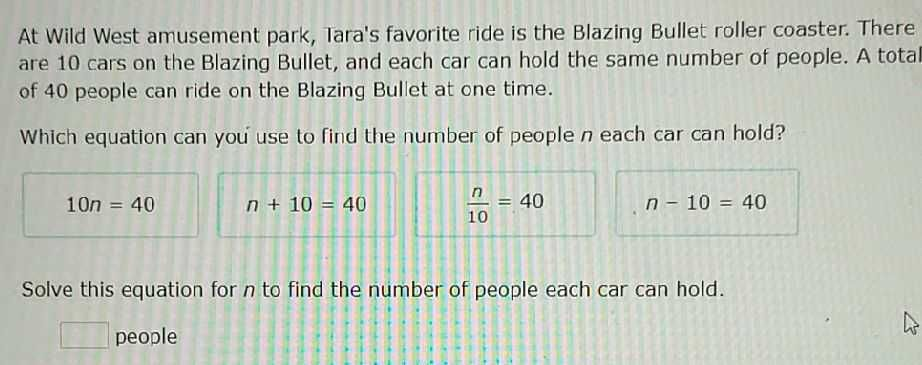### Still have math questions?

Algebra
QuestionAt Wild West amusement park, Tara's favorite ride is the Blazing Bullet roller coaster. There are $$10$$ cars on the Blazing Bullet, and each car can hold the same number of people. A tot of $$40$$ people can ride on the Blazing Bullet at one time.

Which equation can you use to find the number of people $$n$$ each car can hold?

$$10 n = 40$$  $$n + 10 = 40$$     $$\frac { n } { 10 } = 40$$     $$n - 10 = 40$$

Solve this equation for $$n$$ to find the number of people each car can hold.

$$\square$$people

Equation: $$10n = 40$$
$$10n = 40 \\n = 4$$